# AP Statistics : How to use the addition rule

## Example Questions

← Previous 1

### Example Question #1 : Statistical Patterns And Random Phenomena

The probability that it will rain today is 0.35. What is the probability that it will not rain?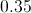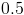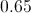Explanation:

The answer is 0.65 because Pr(~Rain) is the complement of Pr(Rain) and both events are mutually exclusive.

When two events are mutually exclusive,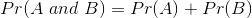. Since probabilities must sum up to 1, this implies that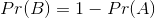### Example Question #1 : How To Use The Addition Rule

Assume there is an election involving three parties: D, R, and I. The probability of D winning is .11, R winning is .78, and I winning is .11. What is the probability of D or R winning?

1

.89

.78

0

.89

Explanation:

Since all of the events are mutually exclusive (one of the parties must win), you can get the probability of either D or R winning by adding their probabilities.

Since the probability of D winning is .11 and R winning is .78, the probability of D or R winning is .89.

### Example Question #1 : Probability

If 1 card is chosen at random from a deck of cards, what is the probability that it will be a heart or a king?

13/52

29/52

17/52

4/13

26/52

4/13

Explanation:

In a deck of cards, there are 52 total cards, 13 hearts, 4 kings, and 1 king that is a heart.

So,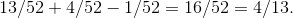### Example Question #1 : Statistical Patterns And Random Phenomena

Using a standard deck of cards, what is the probability of choosing a single face card?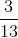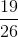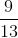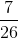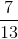Explanation:

There arecards in a standard deck:cards insuits.  There areface cards (King, Queen, Jack) in each suit, so there aretotal face cards.

Thus the probability of choosing a single face card is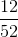or.

### Example Question #1 : Statistical Patterns And Random Phenomena

A student randomly selected a highlighter from her desk.  There were five highlighters on the desk, each of a different color--blue, green, yellow, red, and orange.  What is the probability that the student selected either the red or the yellow highlighter?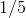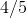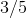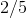Explanation:

In this case, we want to know the probability of multiple, mutually exclusive possible outcomes. To determine the probability of the two possible outcomes, simply add them together.  This is called the addition rule.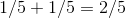### Example Question #1 : How To Use The Addition Rule

What is the probability of obtaining at mostheads when tossing a fair cointimes?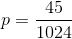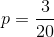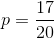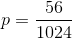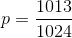Explanation:

First find the number of ways to get 0, 1, or 2 heads.

Remember,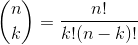.Also,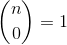and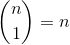.heads: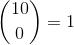head: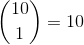heads: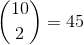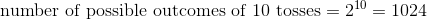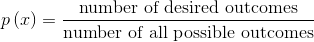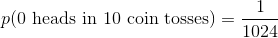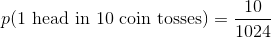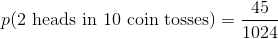Now combine these to find the probability of seeing at most 2 heads in 10 coin tosses: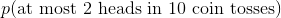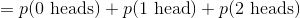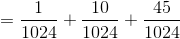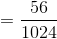### Example Question #1 : Statistical Patterns And Random Phenomena

150 students are athletes at the school. 65 play baseball, 15 play basketball, and 10 play both basketball and baseball.

What is the probability that a randomly selected athlete will play either baseball or basketball or both sports?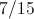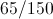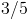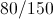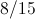Explanation:

We want to know the probability of multiple possible outcomes that are not mutually exclusive. To do this, we use the addition rule with one step that we would not use if the possible outcomes were mutually exclusive.  Add the probabilities of each possible outcome, subtract from that sum the number counted twice, then reduce the answer to the least common denominator.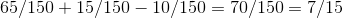### Example Question #1 : Probability

At a business conference, one participant was selected to give a presentation. There weretotal participants.had business degrees anddid not.were entrepreneurs andwere not.of those with business degrees were entrepreneurs. What is the probability that the person selected will have a business degree or be an entrepreneur?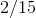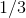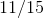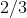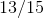Explanation:

We want to know the probability of multiple possible outcomes that are not mutually exclusive. To do this, we use the addition rule with one step that we would not use if the possible outcomes were mutually exclusive.

Add the probabilities of each possible outcome, subtract from that sum the number counted twice, then reduce the answer to the least common denominator. In our case, we are told that 2 of those who have a business degree are also entrepreneurs therefore we need to subtract to from the total to get the correct probability.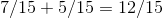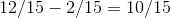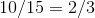### Example Question #1 : Rules Of Probability

In a standard deck ofplaying cards, what is the probability that the first card drawn is a face card (a king, queen or jack) or a diamond?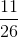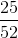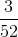Explanation:

Bear in mind how the addition rule applies here. This will be a lengthy application, but applied properly, it will see you through this problem. It helps to think of this as four events that are not mutually exclusive - drawing a queen, drawing a jack, drawing a king or drawing a diamond. We then realize that we have to avoid counting the king, queen and jack of diamonds twice.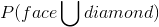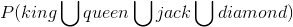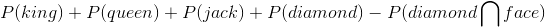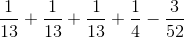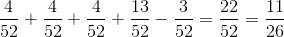### Example Question #2 : Probability

Students collected 150 cans for a food drive.  There were 23 cans of corn, 48 cans of beans, and 12 cans of tomato sauce.  If a student randomly selects one can to give away, what is the probability that the can will be either tomato sauce or beans?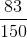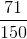Explanation:

In this case, we want to know the probability of multiple, mutually exclusive possible outcomes. The possible outcomes are mutually exclusive because one can of food could not be both beans and tomato sauce. To determine the probability of the two possible outcomes, add them together and then find the least common denominator.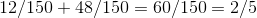← Previous 1

### All AP Statistics Resources